## 3. 4. 2 Topological Neighborhood Considerations

The first step of a finite difference simulation is the determination of the neighborhood. In a very simple two-dimensional case, the neighborhood of a vertex is defined by the four vertices which are covered by the edges incident with the original vertex. It is also assumed that the respective vertex has to fulfill some geometric criterion to be considered as neighboring. In order to obtain all neighboring vertices of a given basis vertex, the traversal function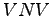is introduced. Figures 3.11 and 3.12 show possible neighborhoods used for the finite difference simulation applied to structured grids and to unstructured meshes.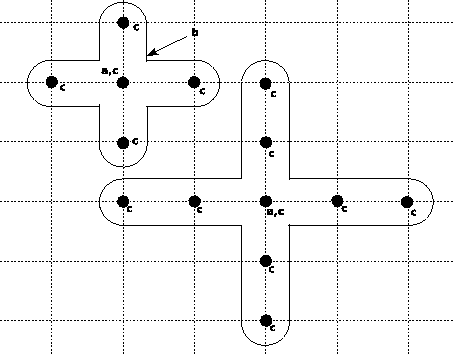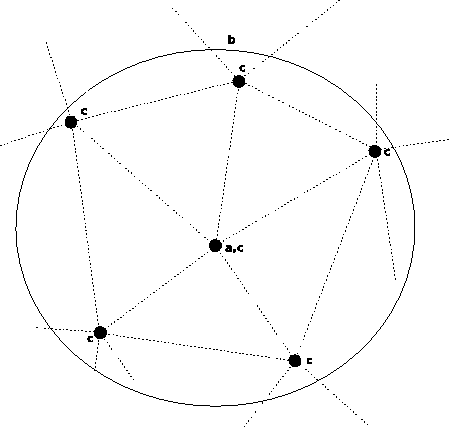For each neighboring vertex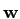of a basis vertex(also the vertexitself, which is within its own neighborhood), the following series expansion is written with respect to the location of the initial vertex: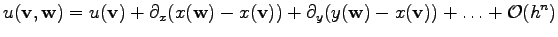(3.52)

For a given truncation error, an appropriate number of neighboring vertices has to be chosen. If a given truncation error is required for a Taylor expansion, for instance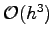, first order and second order terms have to be considered. Each partial derivative of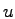as well asitself is considered as solution variable in a coordinate system. If a trunction error of higher order is required, the number of solution variables increases. Accordingly, a larger number of vertices (neighborhood) is required to solve the equation and determine the dependence of the position of the vertices on the value of the derivatives determined.

Moreover, it is possible to determine higher-order derivatives of a function by using a higher-order Taylor expansion. This implies that the use of higher-order derivatives necessarily increases the number of vertices (neighborhood) used for the Taylor expansion.

One obtains a local equation system which can be solved analytically in special cases, especially under the assumption that the local grid intervals are equal (which shall be assumed in the following). One obtains the following equation system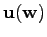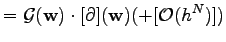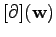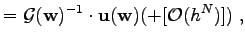(3.53)

where the vector of function values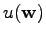is denoted as. The vector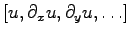is denoted as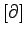evaluated on the vertex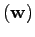. The matrix containing the geometrical coefficients is referred to as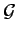. By inverting the geometrical coefficient matrix, one obtains the vector of derivatives. The matrixcan be written as follows:(3.54)

The vector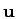can be written as the vector of quantities within the neighborhood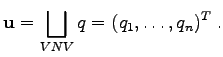(3.55)

A linear differential operator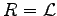can be written as inner product of a given vector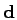and the vector of derivatives.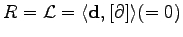(3.56)

By extending the term of canonic partial differential operators, one obtains the residual expression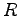. It has to be assured that the order of elements that are passed to the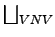operation is the same for the evaluation of the matrixand the vector of function values.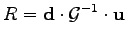(3.57)

Inserting (3.54) without the second order terms and (3.55) into (3.56) yields the following expression.(3.58)

If the geometric constants are known explicitly, the inverse of the matrixcan be derived directly. In this case the matrixcan be written as: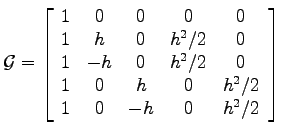The inverse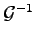of the matrixcan be obtained as follows: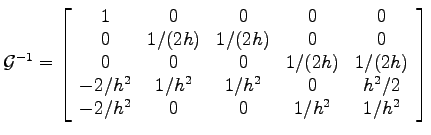The standard finite difference formulae can be obtained easily from the coefficients of the matrix. By the specification of, a linear combination of lines of the matrixis obtained by multiplication. The line vector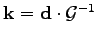denotes the coefficients with which the values of the single neighboring vertices are coupling. It is associated with a vertex and contains coupling coefficients, each of which is directly associated with a neighboring vertex. Alternatively, the vector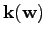can be written element-wise, where the elements are written as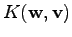. In this casedenotes the vertex anddenotes a vertex in the neighborhood of. By evaluating the inner product of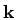with the function values of the single vertices stored in the vector, the residual expression is obtained. The evaluation of the inner product finally yields(3.59)

It can be seen easily that the algebraic structure of (3.59) is similar to other discretization schemes. While other discretization schemes use topologically specified double sums with topological traversal in order to obtain the couplings between solution quantities, the definition of the neighborhood can be defined more freely. While in one case the neighborhood is defined topologically like for finite elements or finite volumes, the neighborhood can also be determined by geometrical considerations.

Michael 2008-01-16